Courses

# Questions:Taylor Series - Mathematical Methods of Physics, UGC - NET Physics Physics Notes | EduRev

## Physics for IIT JAM, UGC - NET, CSIR NET

Created by: Akhilesh Thakur

## Physics : Questions:Taylor Series - Mathematical Methods of Physics, UGC - NET Physics Physics Notes | EduRev

The document Questions:Taylor Series - Mathematical Methods of Physics, UGC - NET Physics Physics Notes | EduRev is a part of the Physics Course Physics for IIT JAM, UGC - NET, CSIR NET.
All you need of Physics at this link: Physics

Problem Set # 1 Multiple Choice Test

COMPLETE SOLUTION SET

1. The coefficient of the x5 term in the Maclaurin polynomial for sin (2 x ) is

(A) 0
(B) 0.0083333
(C) 0.016667
(D) 0.26667

Solution The correct answer is (D).
The Maclaurin series for sin(2 x ) is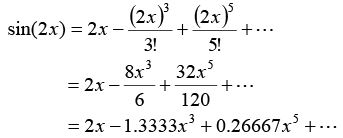Hence, the coefficient of the x5 term is 0.26667.

2. Given f (3) = 6 , f ′(3) = 8 , f ′′(3) = 11 , and all other higher order derivatives of f (x ) are zero at x = 3 , and assuming the function and all its derivatives exist and are continuous between x = 3 and x = 7 , the value of f (7 ) is

(A) 38.000
(B) 79.500
(C) 126.00
(D) 331.50

Solution: The correct answer is (C).
The Taylor series is given by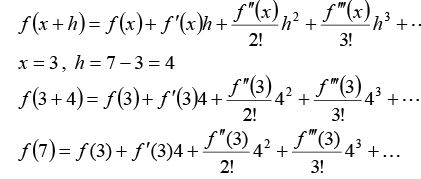Since all the derivatives higher than second are zero,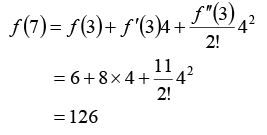3. Given that y(x ) is the solution to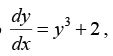y (0) = 3 the value of y(0.2) from a second order Taylor polynomial around x=0 is

(A) 4.400
(B) 8.800
(C) 24.46
(D) 29.00

Solution: The correct answer is (C).
The second order Taylor polynomial is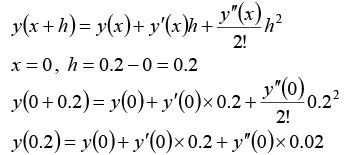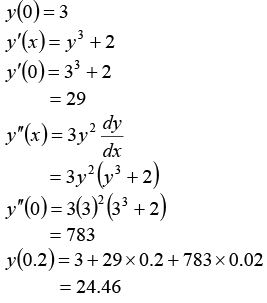4. The series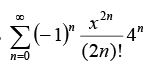is a Maclaurin series for the following function

(A) cos(x)
(B) cos(2 x)
(C) sin (x)
(D) sin (2 x)

Solution: The correct answer is (B).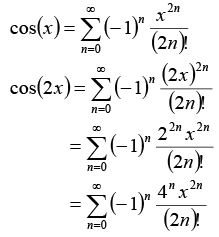5.   The function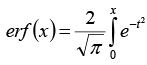dt is called the error function.  It is used in the field of probability and cannot be calculated exactly.  However, one can expand the integrand as a Taylor polynomial and conduct integration.  The approximate value of erf (2.0) using the first three terms of the Taylor series around t = 0 is

(A) -0.75225
(B) 0.99532
(C) 1.5330
(D) 2.8586

Solution: The correct answer is (A).
Rewrite the integral as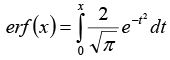The first three terms of the Taylor series for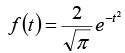around t = 0 are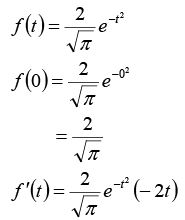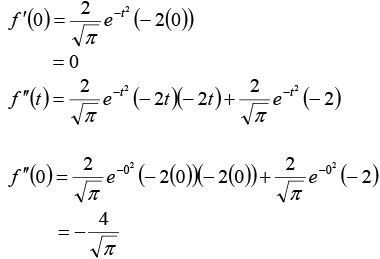The first three terms of the Taylor series are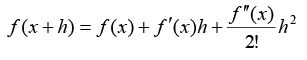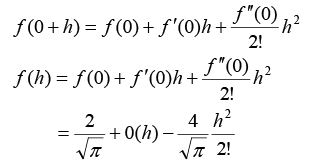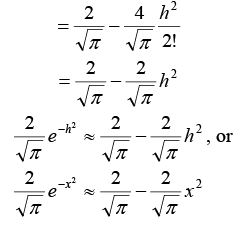Hence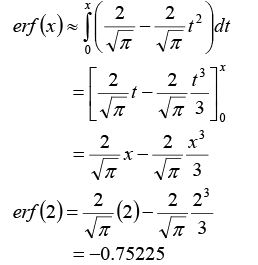Note:  Compare with the exact value of erf (2)

6.  Using the remainder of Maclaurin polynomial of nth order for f (x ) defined as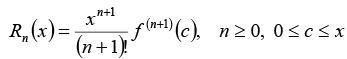the order of the Maclaurin polynomial at least required to get an absolute true error of at most 10 −6 in the calculation of sin (0.1) is (do not use the exact value of sin (0.1) or cos(0.1) to find the answer, but the knowledge that |sin( x)| ≤ 1 and | cos( x) |≤ 1 ).
(A) 3
(B) 5
(C) 7
(D) 9

Solution : The correct answer is (B).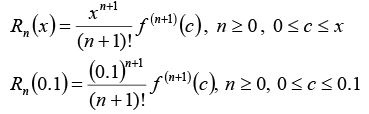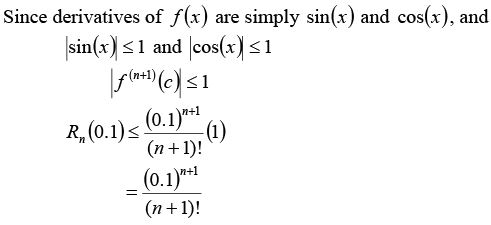So when is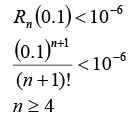But since the Maclaurin series for sin (x ) only includes odd terms, n ≥ 5 .

159 docs

,

,

,

,

,

,

,

,

,

,

,

,

,

,

,

,

,

,

,

,

,

,

,

,

;